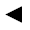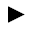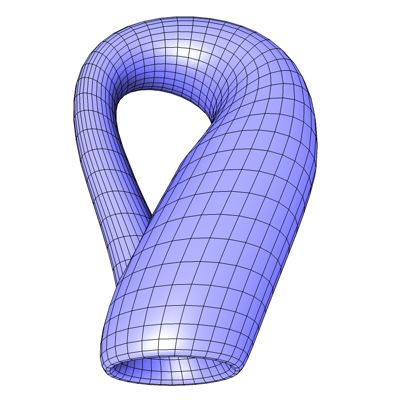п»їп»їп»ї math handbook calculator - Fractional Calculus Computer Algebra System software+++=п»ї[back]

## 3.8. Little Bottle

[next]The so-called Klein bottle [ 6,9,10,11,19 ] was named after the mathematician Felix Klein. It is a three-dimensional extension of the Mцbius strip. Figuratively presented, the Klein bottle is a tube that has been twisted and folded over the fourth dimension in such a way that an object traveling through it ends its journey the wrong way round.

The Klein bottle is represented by the following equations. To keep the formulas a little clearer, we use the constant r.

 r = 4 (1 - cos(u)/2) 3-30

To represent the Klein Bottle, different equations are required for parts of the domain of u.

 0 <= u < pi 3-31 x = a cos(u) (1 + sin(u)) + r cos(u) cos(v) 3-32 y = b sin(u) + r sin(u) cos(v) 3-33 z = r sin(v) 3-34
 pi < u <= 2pi 3-35 x = a cos(u) (1 + sin(u)) + r cos(v + pi) 3-36 y = bsin(u) 3-37 z = r sin(v) 3-38

The constants a and b determine the appearance of the figure.

To represent the area, the two parameters u and v must have the following values ​​(definition range).

 u is an element from the set of numbers [0, 2 pi] v is an element of the number set [0, 2 pi]

Since the Klein Bottle is a closed figure, the definition range must be adhered to exactly, so it cannot be changed with the plugin.
The plugin creates an optimized mesh without duplicate points and unconnected polygons.Fig. 12